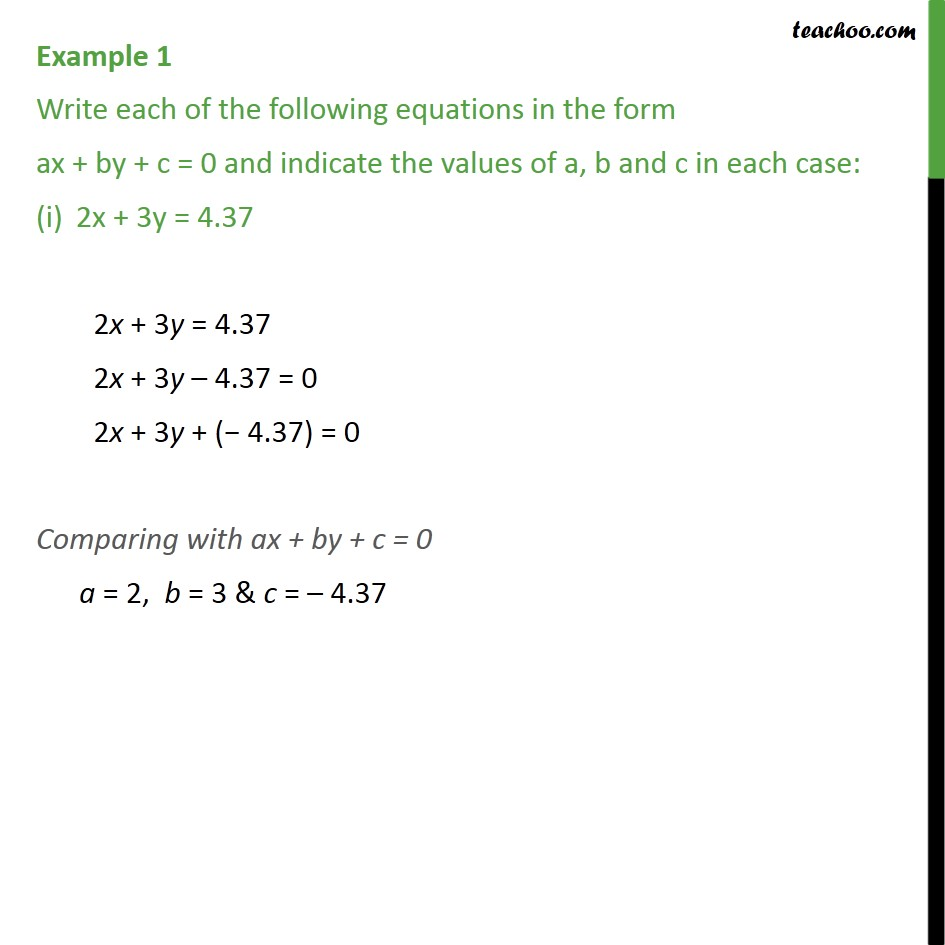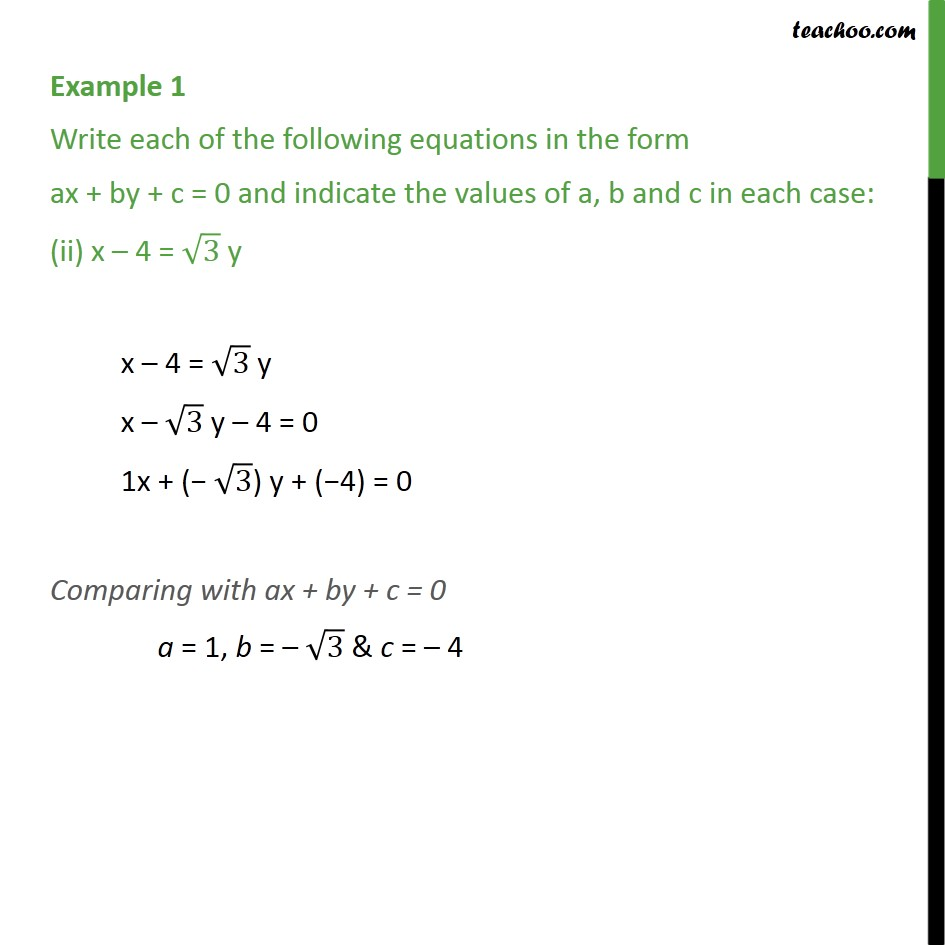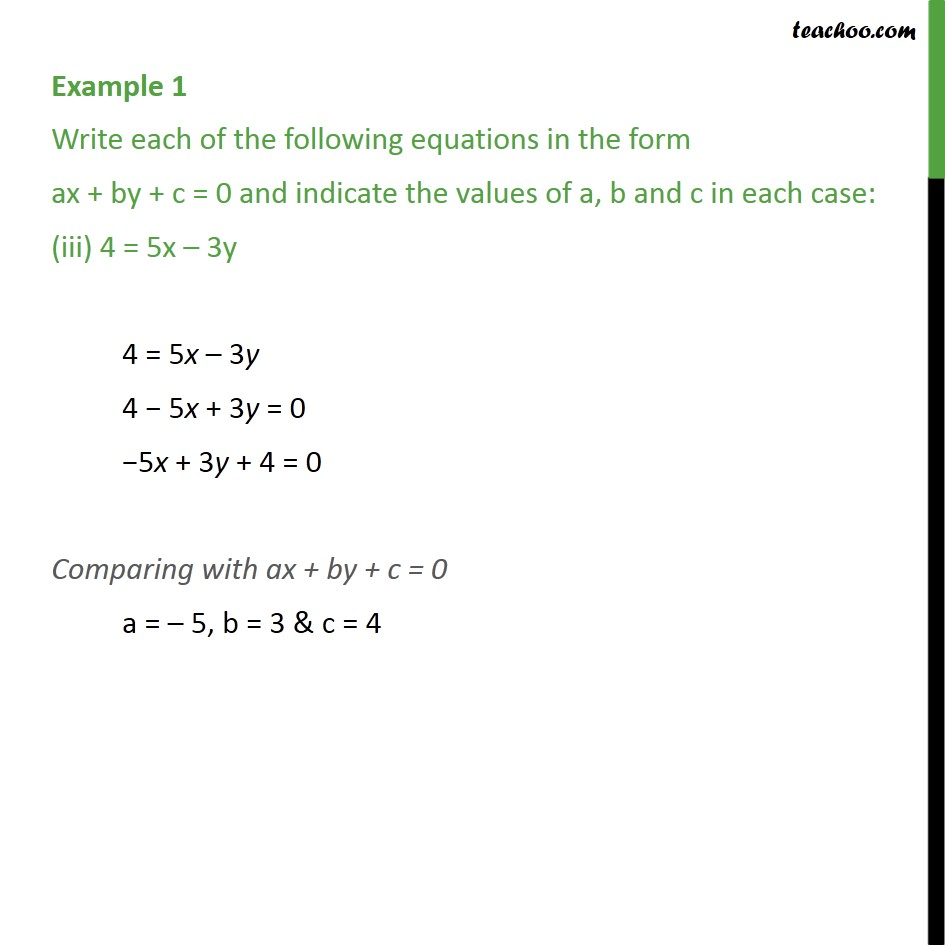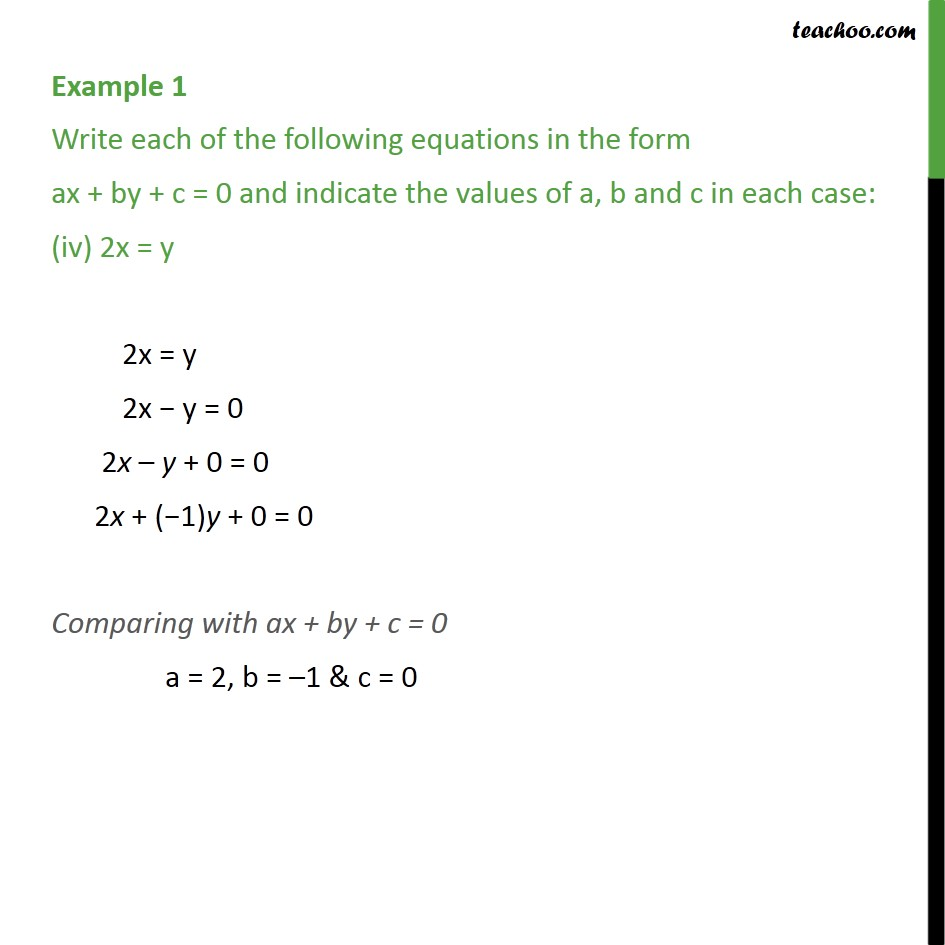Learn all Concepts of Linear Equations Class 9 (with VIDEOS). Check - Linear Equations in 2 Variables Class 91. Class 9
2. Important Questions for Exam - Class 9
3. Chapter 4 Class 9 Linear Equations in Two Variables

Transcript

Example 1 Write each of the following equations in the form ax + by + c = 0 and indicate the values of a, b and c in each case: 2x + 3y = 4.37 2x + 3y = 4.37 2x + 3y – 4.37 = 0 2x + 3y + (− 4.37) = 0 Comparing with ax + by + c = 0 a = 2, b = 3 & c = – 4.37 Example 1 Write each of the following equations in the form ax + by + c = 0 and indicate the values of a, b and c in each case: (ii) x – 4 = √3 y x – 4 = √3 y x – √3 y – 4 = 0 1x + (− √3) y + (−4) = 0 Comparing with ax + by + c = 0 a = 1, b = – √3 & c = – 4 Example 1 Write each of the following equations in the form ax + by + c = 0 and indicate the values of a, b and c in each case: (iii) 4 = 5x – 3y 4 = 5x – 3y 4 − 5x + 3y = 0 −5x + 3y + 4 = 0 Comparing with ax + by + c = 0 a = – 5, b = 3 & c = 4 Example 1 Write each of the following equations in the form ax + by + c = 0 and indicate the values of a, b and c in each case: (iv) 2x = y 2x = y 2x − y = 0 2x – y + 0 = 0 2x + (−1)y + 0 = 0 Comparing with ax + by + c = 0 a = 2, b = –1 & c = 0

Chapter 4 Class 9 Linear Equations in Two Variables

Class 9
Important Questions for Exam - Class 9

About the AuthorDavneet Singh
Davneet Singh is a graduate from Indian Institute of Technology, Kanpur. He has been teaching from the past 9 years. He provides courses for Maths and Science at Teachoo.